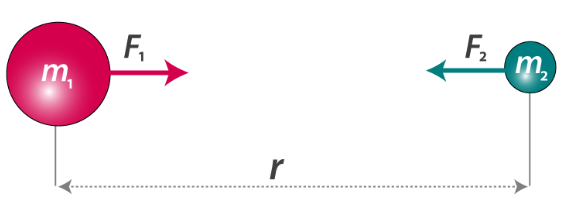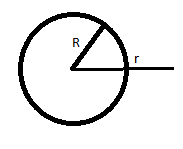# Important Gravitation Formulas for JEE Main and Advanced

Gravitation is the force of attraction between any two objects with mass or energy. This is a natural phenomenon. It is the gravitational force that brings planets, stars and galaxies together. The tides in the ocean are caused due to the gravitational force of attraction of the moon. The weight of physical objects on earth is given as the product of its mass and the acceleration due to the gravity of earth.

1. Gravitational Force

According to Newton’s Law of gravitation, the force between two point masses is given byF= Gm1m2/r2

G is the gravitational constant

The direction of the force will be an attraction

2. Gravitational Potential

i) For Point Charge:

V = -GM/r

ii) For circular ring

$v = \frac{-GM}{\sqrt{R^{2}}+x^{2}}$

iii) For thin circular disc

$v = \frac{-2GM}{R^{2}} (\sqrt{R^{2}+x^{2}}-x)$

iv) Uniform thin spherical shellVout = -GM/r

Vsurface= -GM/R

Vin = -GM/R

3. Gravitational acceleration

g = GM/R2

4. Variation of g with depth

ginside≈ g(1-h/R)

5. Variation of g with height

goutside ≈ g(1-2h/R)

6. Effect of non-spherical earth shape on g

g at pole ＞ g at the equator (since Re – Rp ≈ 21km)

7. Effect of earth rotation on apparent weight

mg’θ = mg = mω2Rcos2θ

8. Orbital velocity of the satellite

$V_{0}=\sqrt{GM/R}$

9. Escape velocity

$V_{e}=\sqrt{2GM/R}$

10. Kepler’s Law

First law: Elliptical orbit with the sun at one of the focus

Second Law: Areal velocity is constant (dA/dt =0)

Third Law: T2 ∝ R3